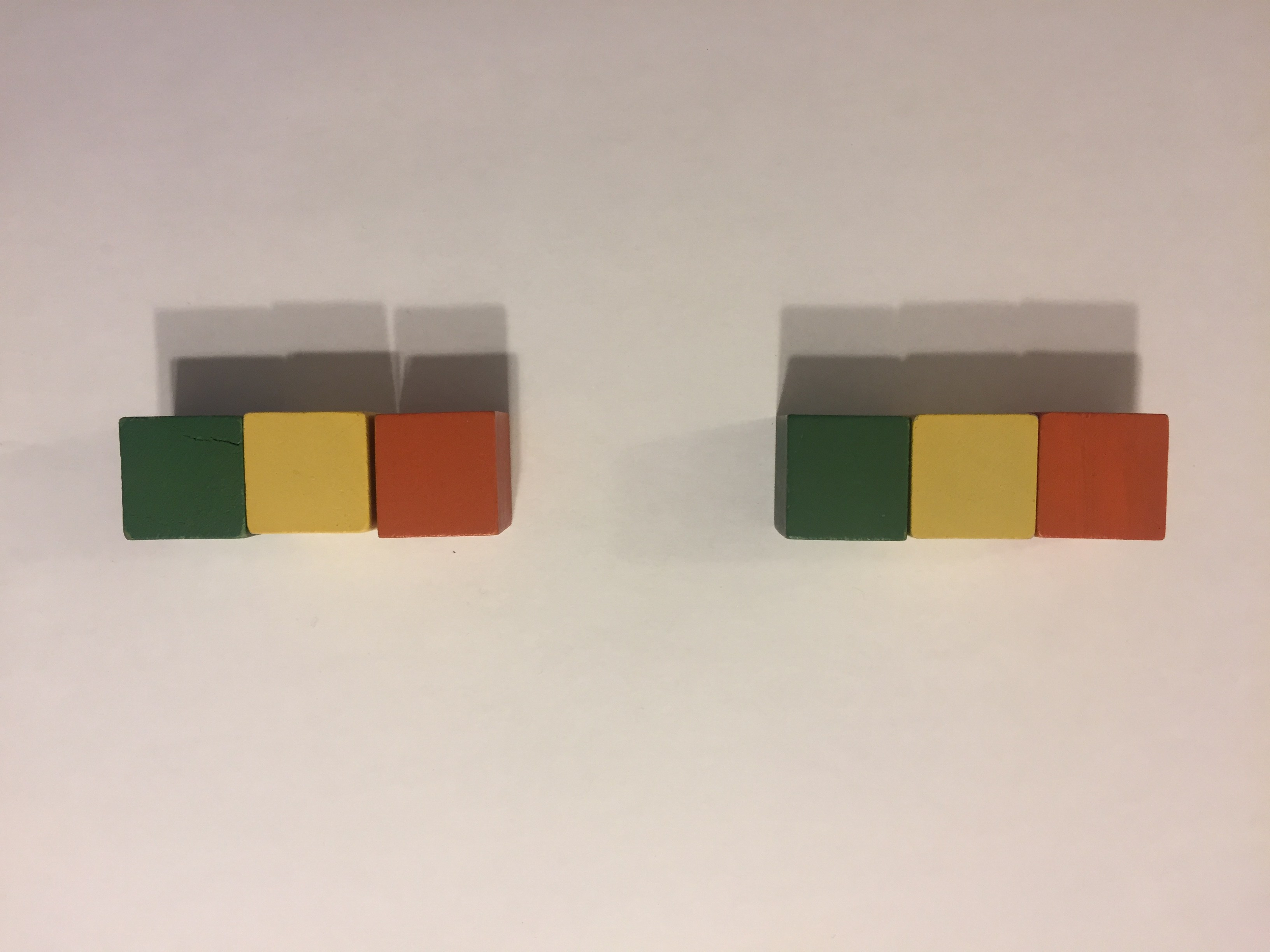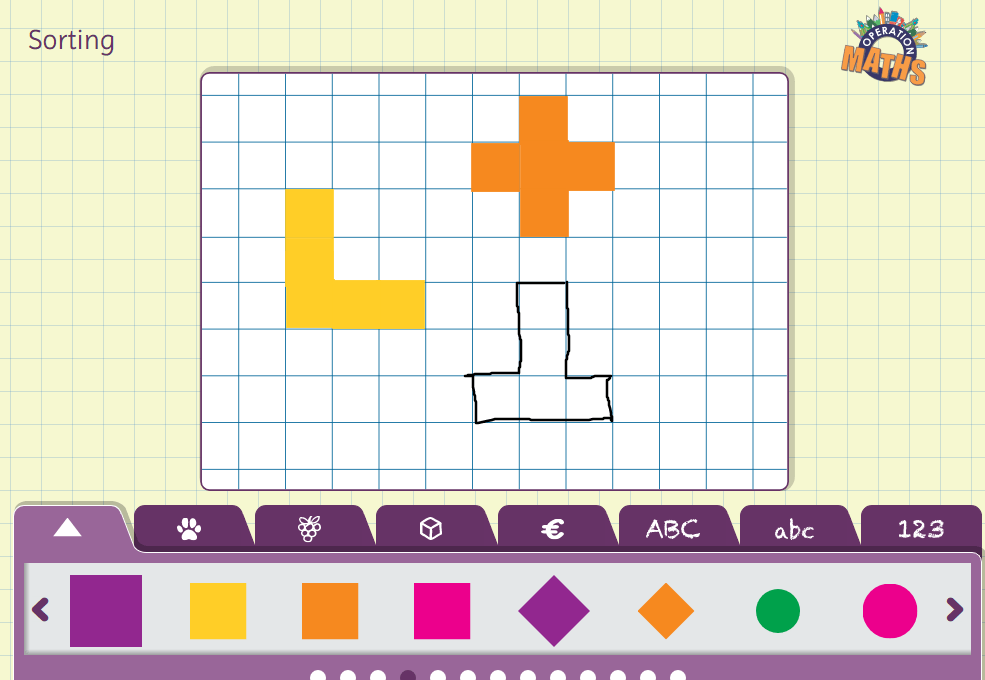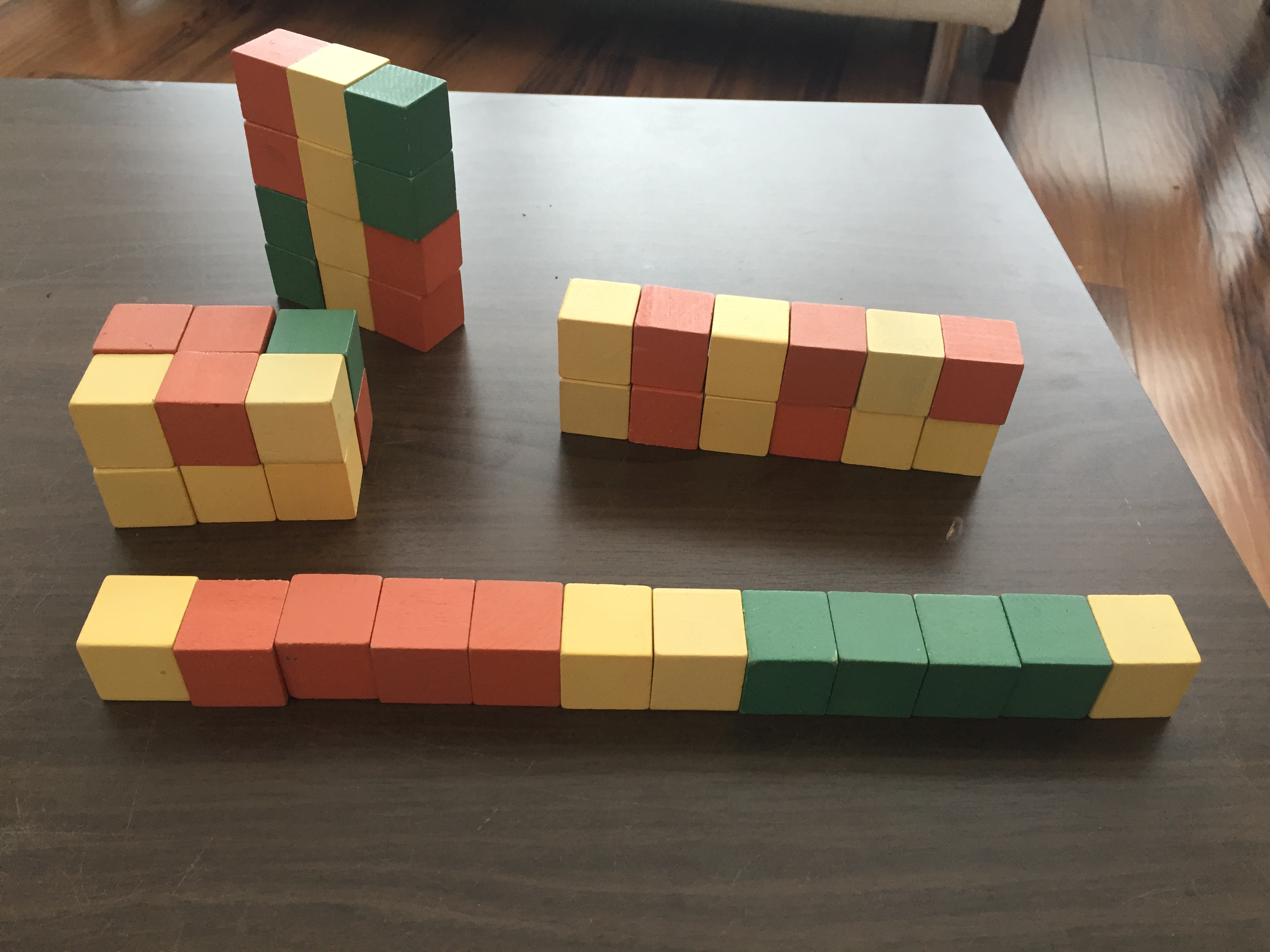# Monthly Archives: March 2018

## Digging Deeper into … Symmetry (2nd to 4th)

Category : Uncategorized

For practical suggestions for families, and links to useful digital resources, to support children learning about the topic of symmetry, please check out the following post: Dear Family, your Operation Maths Guide to Symmetry.

Symmetry is officially a strand unit for second to fourth classes, although it also features as a content objective in 2-D shapes for fifth and sixth class where the children “classify 2-D shapes according to their lines of symmetry”.

While there are different types of symmetry, the curriculum specifies line symmetry, also known as mirror symmetry, reflective or reflection symmetry.

In Operation Maths, this chapter is placed after 2-D shapes, so that the children can identify symmetry in the shapes that they have previously encountered, and, in third  and fourth class, it is placed after Lines and Angles so that they can use their knowledge of different line types when describing the lines of symmetry.

### Concrete exploration

To complete or create symmetrical patterns, requires the children being able to visualise the mirror image of the given arrangement/image. But children cannot visualise what they have not experienced. Thus to experience symmetry the children must:

• be made aware of examples of symmetry all around them, and locate examples themselves e.g. flowers, leaves, objects at home and at school, numbers and letters of the alphabet.
• be afforded ample opportunities to use real mirrors to explore symmetry. The type of child-safe mirrors that are often used in science investigations (eg in the strand unit of light) are ideal for this purpose.Using mirrors allows the children the opportunity to observe symmetry and to check the accuracy of their completed patterns.  When using mirrors:

• Try to have enough mirrors for one between two (the child-safe mirrors can often be cut into smaller sizes, 10cm x 7cm approx is big enough), or if supply is limited the mirror exploration could be incorporated as a station in a station/team teaching maths lesson.
• Initially, allow the children free exploration and then, when suitable, guide it towards a purpose using questioning:
• What letters or numbers look the same in the mirror? What shapes or images in the environment look the same in the mirror?
• Can you put the mirror along the middle of any shapes and numbers so that they look complete? Does this work with any shapes or images from the environment? Don’t specify “middle” as being horizontal or vertical, and then see if the children realise that, on some figures, there is more than one than one way that the mirror can be placed.
• At this point you could use this as the introduction to a separate and distinct What do you notice? What do you wonder? activity, and use the children’s wonder questions to guide the course of the rest of the lesson.
• Explain that, on the symmetrical figures, the position of the mirror, is referred to as the line of symmetry. Then ask the children to use the mirrors to identify/draw the line of symmetry on the figures or mark the line of symmetry first (more challenging) and then check using the mirror.
• Using the mirrors the children can create and check symmetrical patterns using cubes, counters, objects etc. One child can create a pattern that their partner has to complete symmetrically. Since children often incorrectly replicate the pattern (eg as done in the first image below) rather than reverse it, the mirror can show them their error (as used in second image below). Encourage the children to realise that whatever is closest to the mirror/line of symmetry on one side will also be closest to the mirror on the other side.• The children could then progress to creating symmetrical arrangements of more than one row. The Operation Maths twenty frames (free with Operation Maths 1 and 2) can be very useful for this (see below). Again the children should be encouraged to recognise that the colour and type of object/figure that is closest to the line of symmetry on one side should also be closest to the line of symmetry on the other side.

When the children have had sufficient experience with actual mirrors they should progress to completing activities without them, although they could always be returned to again if needs arose.

## Digging Deeper into … Area (2nd to 6th)

Category : Uncategorized

For practical suggestions for families, and links to useful digital resources, to support children learning about the topic of area, please check out the following post: Dear Family, your Operation Maths Guide to Area

When most of us think of area, we probably think of Area = Length x Width. And this in itself hints at the difficulties with this topic; our knowledge of area often centers around a formula rather than understanding the concept of area (and the ability to visualise area) as the amount of space that a surface covers/takes up (as defined in the Maths Dictionary for Kids).

Area is introduced in Operation Maths 2. Initially, the children are enabled to consider space on a surface and which has the greater area (covers more) or the lesser area (covers less) as shown below.In Operation Maths:

• Area is taught after 2-D Shapes as the children will need to use their knowledge of the properties of 2-D shapes and tessellating patterns to appreciate which shapes are best to accurately cover a surface.
• Area is taught after Length as, from 4th class up, the children require previous experience of measuring the length of an object/figure.
• Area is also taught after Length in 4th class up, so as to avoid the children meeting both area and perimeter, initially, at the same time. That said, once it appears that the children have grasped the concept of area as the size a surface covers, then the connections with perimeter should be explored (see more on this below)
• Because, in Operation Maths 6, the chapter on Area conveniently follows on from the chapter on Length, this also allows children to measure/calculate areas on room plans using their knowledge of scale, introduced in the Length chapter. This can be extended by the children measuring the dimensions of a specified area in the school grounds, e.g. pitch, car park and drawing a plan of the area to different scales.

### Measuring area

Measuring area means to establish the area of a shape by measuring and/or counting the number of square units required to cover it (or it covers, when laid on top). Initially in second class, and as revision in third class, the children will be exploring this using non-standard units that are both square and non-square, for example playing cards, envelopes, etc. Through this exploration, it is hoped that the children will come to the realisation that it is preferable to use a standard square unit.At this initial measuring phase, the children should be given as many opportunities as possible to measure the area of both regular and irregular shapes. These experiences could include:

• Making shapes on a geoboard with elastic bands and measuring the area within; this can be modeled also on this online interactive geoboard
• Placing transparent/translucent shapes on a grid to count the square units covered by the shape. Progress to using opaque shapes, as these are more challenging. The Operation Maths Sorting eManipulative can also be used to model this (see image below)
• Make shapes that have the same area but look different. To do this, give the children  opportunities to draw different shapes of equal area on squared paper; “same area value, different appearance”. Again, this can be modeled, as shown below, using the Operation Maths Sorting eManipulative.• In the senior classes, square tiles, unifix cubes and/or the units in base ten blocks  can be used to link the concept of “same area value, different appearance” to both the area model of multiplication and identifying the various factor pairs for a number as shown in Number Theory. For example, the children can make rectangles of various dimensions, but all with an area of 36, and thus they can identify that the the factors of 36 are 1 x 36, 2 × 18, 3 × 12, 4 × 9 and 6 × 6.

In Operation Maths 3, by using squared paper/grids the children are introduced to using a standard square unit for measuring area. If the squared paper/grids are also centimeter grids this leads logically on to work in 4th class, where this square unit is then identified specifically as a square centimetre.

### Estimation and efficiency

When using both non-standard and, later, standard square units, the children should always be encouraged to estimate the area first before measuring. As mentioned previously in the post on Length, rather than estimating the area of A, B, C and D before measuring A, B, C and D, it would be better if the children estimated the area of A and then measured/counted the area of A, estimated the area of B and then measured/counted the area of B and so on. Thus, they can reflect on the reasonableness of their original estimate each time and use this to refine their next estimate so that it might be more accurate. In this way, the children will also begin to develop their sense of space.

Some shapes may cover only parts of squares and this allows for opportunities to discuss what strategy to use to count these, for example two half squares count as one, less than half a square does not count, more than half a square counts as one.

As the children’s understanding develops, they should also be encouraged to come up with increasingly more efficient strategies for measuring area:

• “How did you find out the area of the rectangle?”
• “Did you count the squares?”
• “Is there a faster (more efficient way) to count the squares rather than counting them in ones? Explain. “

Allow the children to verbalise and explain their strategies, as this discussion will likely reveal approaches that incorporate aspects of repeated addition and/or multiplication, thus leading on well to the children deducing a method to calculate area.

### Calculating area

The children begin to calculate area as opposed to measuring (counting area) in 5th  class. However, this should not be introduced purely with the introduction of the formula for calculating the area of a rectangle, rather, as mentioned above, it is hoped that though sufficient opportunities of counting squares in previous classes that the children will now suggest more efficient strategies, including repeated addition and multiplying the length by the width. Considering also, that Operation Maths regularly uses the visual image of rectangular arrays to model multiplication (referred to as the area model), these experiences in multiplication will prepare the children well for the concept of calculating area via multiplication.

Initially, it is preferable that the children are calculating the area of shapes that can be easily checked by measuring. Then, when ready, they should progress to calculating area using more abstract measures such as millimeters, ares and large numbers of metres. They can also apply their knowledge to calculating the area of other shapes (eg triangles) and to irregular shapes that can be easily partitioned into rectangles (often referred to as compound shapes).  Finding the area of a circle (6th class) is by counting squares only and is covered in the chapter on the Circle.

### Area and perimeter

As mentioned above, to avoid confusion between the concepts of area and perimeter, it is important that they are both taught separately, initially. The concept of perimeter as the length around the outside of a shape is not introduced until 4th class, meaning that in 2nd class and 3rd class the children can just explore the concept of area, without the confusion of adding perimeter to the mix!

When ready, the children can begin to explore the connections between the two concepts. And it is essential that both concepts are taught, using a visual context e.g.:

• fences (perimeter) and sheep/grass (area)
• skirting boards (perimeter) and tiles/carpet (area)
• fences (perimeter) and stone slabs (area)
• or any other context with which the children might be most familiar (see also the video at the end with shows the both concepts in various contexts)

The children can build models and/or draw outlines to represent area and perimeter:

• make a fence using lollipop sticks or match sticks on large sheets of paper and sketch the square units within the border to match the length of each unit of “fence”.
• Place the units from base ten blocks on a centrimetre square grid as sheep and draw units of fencing around them. Or use unifix cubes to do the same but on 2cm square grids as unifix cubes are 2cm long on each side.

Through this exploration, it is likely that the children will begin to realise that the perimeter of a rectangular shape does not determine the area of the shape. Using the concrete materials allow the children to construct both rectangles of constant area but varying perimeter and rectangles of constant perimeter with varying areas and to help develop the concept. Again, use a context if possible to reinforce the two concepts:

• A farmer wants to build a sheep enclosure for 12 sheep, giving each sheep one square unit of space (use base ten units or cubes, as shown below). Show three different ways this could be done. Which way requires the most fencing? Which requires the least fencing?
• A different farmer has 24 units of identical fencing. Show three different ways the fencing could be arranged. Which arrangement can take the most sheep, giving each sheep one square unit of space? Which arrangement can take the least sheep?Some of the children may discover that the most efficient use of fencing, to produce the largest area, will be a square shape or a shape closest to a square, if not possible to make a square. In a similar way, in 6th class, when the children begin to investigate surface area, the children can investigate how the volume of a shape does not determine the surface area of the shape. They could use the base ten units (or any other available cubes) to build cubes/cuboids with the same volume (eg 12, 18, 24 etc cubic units), but in different arrangements each time, and measure (count)/calculate the surface area of each resulting arrangement.